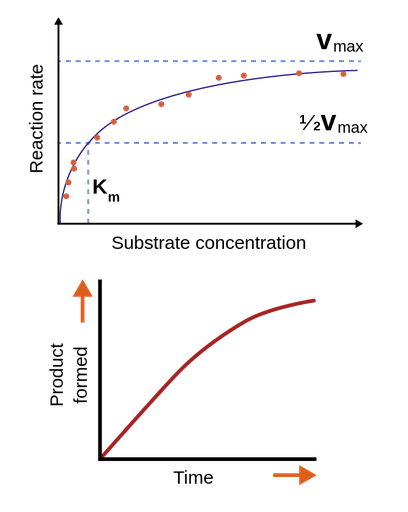Enzyme kinetics is the study of enzyme mechanisms through determination of reaction rates under varied conditions. The rate of a reaction is dependent on several factors including the concentration of the substrate and the enzyme, temperature, pH and presence of inhibitors.Figure 1: Top: Michaelis-Menten saturation curve. Bottom: Progress curve of a general enzymatic reaction.

## Performing kinetic assays

The aim of a kinetic assay is to model the reaction rate, V, as a function of the substrate concentration, [S], as illustrated in Figure 1. It is, therefore, necessary to measure the rate at several different substrate concentrations. For each substrate concentration, a progress curve showing the amount of product formed as a function of time is obtained. Such a progress curve is illustrated in Figure 1. As the figure shows, the rate of the reaction is approximately constant early in the reaction; however, as the substrate is used up, the rate decreases, and the progress curve reaches a plateau. Because [S] changes during the reaction, it is common to measure the initial rates (V0) of the reactions and plot these against the substrate concentration. V0 is measured as the slope of the progress curve at the beginning of the reaction when the progress curve is still linear .

The simplest model of V as a function of [S] is the Michaelis-Menten equation. Reactions where the Michaelis-Menten equation can be applied, show an increase in the reaction rate when [S] is increased; however, this increase is diminished as the rate approaches the maximum velocity, Vmax as seen in Figure 1. The Michaelis-Menten equation will be further described on the following pages .

## Factors affecting the reaction rate

Temperature and pH also affect the reaction rate. Enzymes have an optimum pH, which is dependent on the composition of the enzyme. This is due to the properties of the amino acid side chains of the enzyme: some side chains need to be protonated or deprotonated in order to make a functional enzyme. Whether an amino acid side chain is protonated or deprotonated depends on the pKa of the side chain and the pH of the solution. For instance, histidine has a pKa of 6.0, which means that it will be mostly protonated at pH<6.0 and mostly deprotonated at pH>6.0. Note that the pKa of side chains may be altered by the environment; therefore the pKa of the side chains in enzymes is usually not the same as of the free amino acids. Temperature also affects the reaction rate: an increase in temperature leads to an increased reaction rate; however, at a certain temperature, depending on the enzyme, the enzyme will start to denature; therefore, the reaction rate will start decreasing above this temperature .

## The simulator

In this case, you will use a simulator to perform the kinetic experiments. This simulator is based on a mathematical model, and it is therefore "perfect", i.e., it does not result in any experimental errors. This is, of course, not how the outcome of an enzyme kinetic assay would work in real life, where such perfect data would not be obtainable.

1. Lehninger, Albert L.; Nelson, David L.; Cox, Michael M. (2008). Principles of Biochemistry (5th ed.). New York, NY: W.H. Freeman and Company. ISBN 978-0-7167-7108-1.

Theory overview